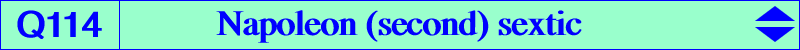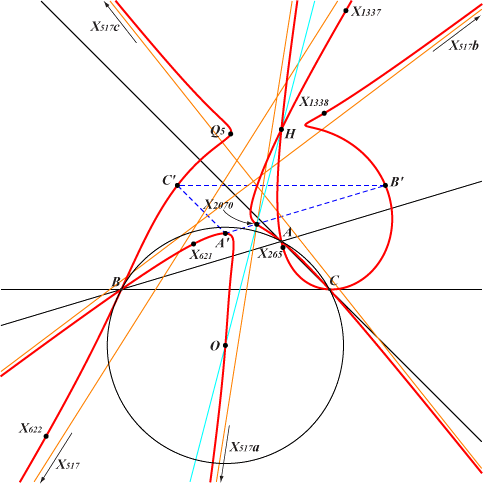too complicated to be written here. Click on the link to download a text file.X(3), X(4), X(265), X(517), X(621), X(622), X(1337), X(1338), X(2070) extraversions of X(517) A', B', C' : reflections of X(3) in the sidelines of ABC (Carnot / Johnson triangle)Q114 is defined in the page K005, see property 7. Q114 is a circular sextic with four real asymptotes whose infinite points are X(517) and extraversions. The asymptote passing through X(517) contains X(119), the antipode of X(11) on the nine point circle. The remaining imaginary asymptotes meet at the singular focus F which does not lie on the curve. H = X(4) is a quadruple point and A, B, C are double points. Any point P ≠ H on K005 is transformed into a point Q on Q114 such that Q = H-agP /\ O-gagP where a, g denote anticomplement and isogonal conjugate as usual. This is related to CL037. When P = X(5), we obtain Q5 on the lines X(4)X(93), X(5)X(252), X(30)X(930), etc, with SEARCH = -3.50663728517664. This is X(19552) in ETC.